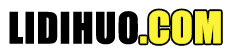Makefile教程# Makefile 教程

Makefile 是一种程序构建工具，可在 Unix、Linux 及其风格上运行。它有助于简化可能需要各种模块的程序可执行文件的构建。为了确定模块需要如何编译或重新编译，make需要用户定义的 makefile 的帮助。本教程将增强您对 makefile 的结构和实用程序的了解。

Makefile 是特殊格式的文件，可帮助自动构建和管理项目。

main.cpp hello.cpp factorial.cpp functions.h
main.cpp

```#include <iostream>
using namespace std;
#include "functions.h"
int main(){
print_hello();
cout << endl;
cout << "The factorial of 5 is " << factorial(5) << endl;
return 0;
}
```
hello.cpp

```#include <iostream>
using namespace std;
#include "functions.h"
void print_hello(){
cout << "Hello World!";
}
```
factorial.cpp
factorial.cpp 的代码如下-
```#include "functions.h"
int factorial(int n){

if(n!=1){
return(n * factorial(n-1));
} else return 1;
}
```
functions.h

```void print_hello();
int factorial(int n);
```

```gcc  main.cpp hello.cpp factorial.cpp-o hello
```

make 命令允许您管理大型程序或程序组。当您开始编写大型程序时，您会注意到重新编译大型程序比重新编译短程序需要更长的时间。此外，您会注意到您通常只处理程序的一小部分（例如单个函数），而其余程序的大部分都没有改变。